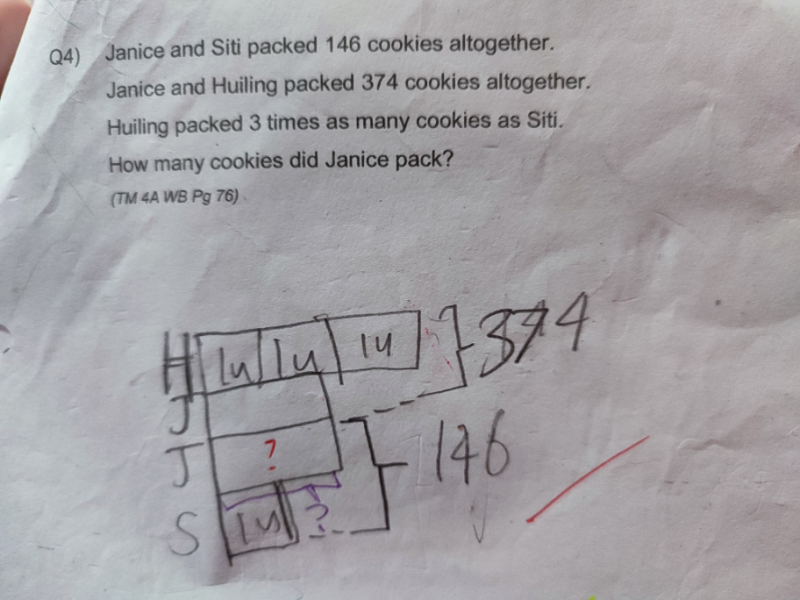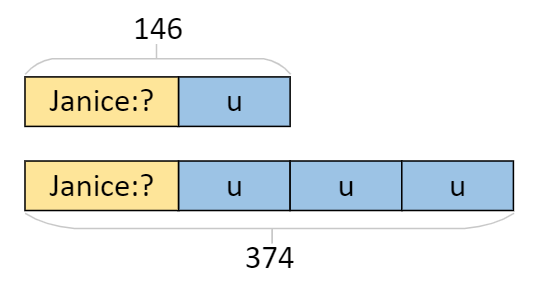# QuestionHi able to write model. Unable to solve. Kindly help

```You are almost there with your diagram!

Redraw the boxes horizontally:``````Then 2u = 374 - 146 = 228. So u = 228/2 = 114.
Thus Janice packed 146 - 114 = 32 cookies.

CHECK:
Janice + Siti    = 32 + 114 = 146
Janice + Huiling = 32 + 3 x 114 = 374

Btw, you misclassified this question as P4 MALAY, instead of MATH.```
0 Replies 2 Likes ✔Accepted Answer